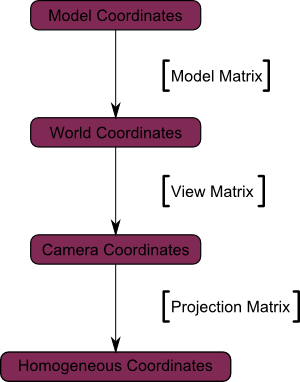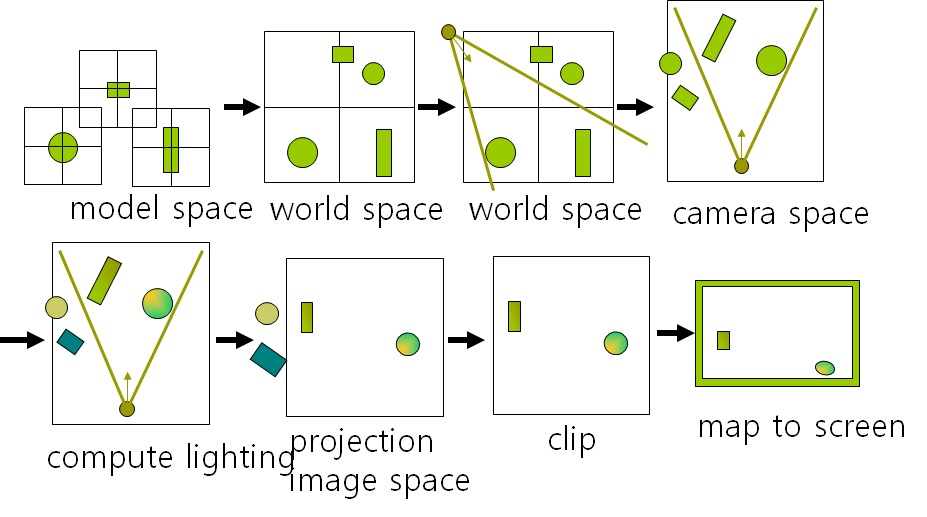## The Model matrix

This model, just as our beloved red triangle, is defined by a set of vertices. The X,Y,Z coordinates of these vertices are defined relative to the object’s center : that is, if a vertex is at (0,0,0), it is at the center of the object.

We can sum this up with the following diagram :## The View matrix

We went from World Space (all vertices defined relatively to the center of the world, as we made so in the previous section) to Camera Space (all vertices defined relatively to the camera).

Here’s the compulsory diagram :## The Projection matrix

We’re now in Camera Space. This means that after all theses transformations, a vertex that happens to have x==0 and y==0 should be rendered at the center of the screen.

And the final diagram :## Coordinate System## HW1

각자 lab1 & lab2를 사용하여 본인만의 2D & 3D primitive 그래픽을 만들어온다. (Due by 9/30)

## Project Idea Presentation

Project Idea Presentation (9/30) 프로젝트 제목, 내용, HW/SW

1. 안효나

2. 한병휘, 박지환

3. 남호철, 문성현

4. 장원준, 현지웅

5. 김준우, 차인규

6. 손의성, 양채은

7. 서덕화, 김의성